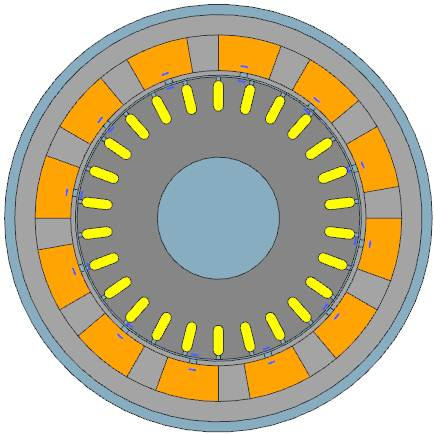# Induction Motor Parameter IdentificationThe example shows how to determine and optimize the performance of a test induction (asynchronous) machine.

Download as PDF

Of particular interest is the method that is used for parameter identificationinductance and ohm resistors are calculated with FEWith these values, an analytical network statement is fed.

## Initial Situation

### Induction Machine

We want to use the Magnetics program to determine the performance of a test induction machine at speed and under load. For this, we want to determine the following characteristic graphs of the engine:

- Torque over Revolution Speed,
- Efficiency over Revolution Speed,
- Current over Revolution Speed.Picture: CAD Model of Motor

Some of the characteristics of this motor are:

- Stator number of Slots: 12
- Stator outside diameter: 400 mm
- Rotor number of conductors: 26
- Thickness: 162 mm
- Number of poles: 2

The methods shown here base on the following literatures:
Calculation of two-axis Induction Motor Model Parameters using Finite Elements
- and David Meekers "Induction Motor Example".

## Appropriate Method

### Combined Analytical / FEM

Rather than modeling the machine’s behavior directly (we could do this through transient analysis), we will infer the performance at speed and load through a series of simulations of static configurations. From these simulations we will derive the equivalent circuit parameters of the machine that we will further use in an analytic electric circuit model. The advantage of an analytic circuit mode is that it allows very fast analysis of different parameters.

A circuit model of one phase for an asynchronous motor is displayed in the following picture.Picture: Steady State Induction Motor Model

L: Inductance of all leakage on the stator side
M: Inductance of the magnetic circuit linking the rotor and stator
Rr: Rotor ohm resistance
Rs: Stator ohm resistance
V: Phase voltage, RMS voltage applied across each phase
i: Phase current, RMS current through each phase of the machine
ω: Electrical frequency
ωs: difference between the rotor’s mechanical frequency and its electrical frequency.

Through finite element analysis it is possible to compute coil inductances with a high accuracy. This is a very helpful feature because it allows to use them in analytical formulas that describe the circuit. In our case we derive the needed inductance values in the following way: We perform a frequency sweep analysis over 12 low frequencies.

As result we get real and imaginary parts for 12 inductances. We run these values through an implemented parameter fitting algorithm and get as result the fitted values for L1, M, Rr :

L  = 34,6 mH     Stator leakage inductance
M = 139,5 mH   Mutual inductance between rotor and stator
Rr = 0,31 Ohm  Room temperature rotor resistance

Next we adapt the rotor resistance values to 60°C temperature increase and we also compute the stator ohm resistance to Rr = 1 Ohm

## Result

### Torque, Efficiency, Current

All these data, resulting from the FE and analytics, we store in a clearly arranged Mathcad document. This allows the user in a convenient manner to understand the formulas used and manipulate them.

In the document, the formulas are evaluated, resulting from the circuit model. The result is the characteristic graphs that describe the motor. Now the engine can be evaluated.Picture: The torque curve shows the characteristic behaviour of an asynchronous motorPicture: The curve showing efficiencyPicture: The current of the motor

Another advantage is the automatic updating of the graph when you change the input parameters. Thus, if the motor parameters are changed, it can immediately be identified whose impact on the graph. In this way it is easy to suitably adjust the motor and adapt it to requirements.